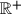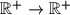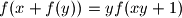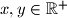### MEMO 2012 pojedinačno problem 1

Kvaliteta:
Avg: 4,3
Težina:
Avg: 7,0
Let$\mathbb{R} ^{+}$ denote the set of all positive real numbers. Find all functions$\mathbb{R} ^{+} \to \mathbb{R} ^{+}$ such thatholds for all$x, y \in \mathbb{R} ^{+}$.
Izvor: Srednjoeuropska matematička olimpijada 2012, pojedinačno natjecanje, problem 1